SNVS691H January   2011  – October 2015

PRODUCTION DATA.

1. Features
2. Applications
3. Description
4. Revision History
5. Pin Configuration and Functions
6. Specifications
7. Detailed Description
1. 7.1 Overview
2. 7.2 Functional Block Diagram
3. 7.3 Feature Description
4. 7.4 Device Functional Modes
8. Application and Implementation
1. 8.1 Application Information
2. 8.2 Typical Application
1. 8.2.1 Design Requirements
2. 8.2.2 Detailed Design Procedure
1. 8.2.2.1 Design Steps for the LMZ14202H Application
3. 8.2.3 Application Curves
9. Power Supply Recommendations
10. 10Layout
11. 11Device and Documentation Support
12. 12Mechanical, Packaging, and Orderable Information

• NDW|7

## 6 Specifications

### 6.1 Absolute Maximum Ratings

over operating free-air temperature range (unless otherwise noted)(1)(2)(3)
MIN MAX UNIT
VIN, RON to GND –0.3 43.5 V
EN, FB, SS to GND –0.3 7 V
Junction Temperature 150 °C
Peak Reflow Case Temperature
(30 s)
245 °C
Storage Temperature –65 150 °C
(1) Stresses beyond those listed under Absolute Maximum Ratings may cause permanent damage to the device. These are stress ratings only, which do not imply functional operation of the device at these or any other conditions beyond those indicated under Recommended Operating Conditions. Exposure to absolute-maximum-rated conditions for extended periods may affect device reliability.
(2) If Military/Aerospace specified devices are required, please contact the Texas Instruments Sales Office/ Distributors for availability and specifications.
(3) For soldering specifications, refer to the following document: SNOA549

### 6.2 ESD Ratings

VALUE UNIT
V(ESD) Electrostatic discharge Human body model (HBM), per ANSI/ESDA/JEDEC JS-001(1) ±2000 V
(1) JEDEC document JEP155 states that 500-V HBM allows safe manufacturing with a standard ESD control process.

### 6.3 Recommended Operating Conditions

over operating free-air temperature range (unless otherwise noted)
MIN MAX UNIT
VIN 6 42 V
EN 0 6.5 V
Operation Junction Temperature −40 125 °C

### 6.4 Thermal Information

THERMAL METRIC(1) LMZ14202H UNIT
NDW (TO-PMOD)
7 PINS
RθJA Junction-to-ambient thermal resistance 4-layer Printed-Circuit-Board, 7.62 cm × 7.62 cm (3 in × 3 in) area, 1-oz Copper, No air flow 16 °C/W
4-layer Printed-Circuit-Board, 6.35 cm × 6.35 cm (2.5 in × 2.5 in) area, 1-oz Copper, No air flow 18.4
RθJC(top) Junction-to-case (top) thermal resistance No air flow 1.9 °C/W

### 6.5 Electrical Characteristics

Limits are for TJ = 25°C unless otherwise specified. Minimum and Maximum limits are ensured through test, design or statistical correlation. Typical values represent the most likely parametric norm at TJ = 25°C, and are provided for reference purposes only. Unless otherwise stated the following conditions apply: VIN = 24 V, VOUT = 12 V, RON = 249 kΩ
PARAMETER TEST CONDITIONS MIN(1) TYP(2) MAX(1) UNIT
SYSTEM PARAMETERS
ENABLE CONTROL
VEN EN threshold trip point VEN rising 1.18 V
over the junction temperature (TJ) range of –40°C to +125°C 1.1 1.25
VEN-HYS EN threshold hysteresis 90 mV
SOFT-START
ISS SS source current VSS = 0 V 10 µA
over the junction temperature (TJ) range of –40°C to +125°C 8 15
ISS-DIS SS discharge current -200 µA
CURRENT LIMIT
ICL Current limit threshold DC average 3.2 A
over the junction temperature (TJ) range of –40°C to +125°C 2.4 3.95
VIN UVLO
VINUVLO Input UVLO EN pin floating
VIN rising
3.75 V
VINUVLO-HYST Hysteresis EN pin floating
VIN falling
130 mV
ON/OFF TIMER
tON-MIN ON timer minimum pulse width 150 ns
tOFF OFF timer pulse width 260 ns
REGULATION AND OVERVOLTAGE COMPARATOR
VFB In-regulation feedback voltage VIN = 24 V, VOUT = 12 V
VSS >+ 0.8 V
TJ = -40°C to 125°C
IOUT = 10 mA to 2 A
0.803 V
over the junction temperature (TJ) range of –40°C to +125°C 0.782 0.822
VIN = 24 V, VOUT = 12 V VSS >+ 0.8 V
TJ = 25°C
IOUT = 10 mA to 2 A
0.786 0.803 0.818
VFB In-regulation feedback voltage VIN = 36 V, VOUT = 24 V
VSS >+ 0.8 V
TJ = -40°C to 125°C
IOUT = 10 mA to 2 A
0.803 V
over the junction temperature (TJ) range of –40°C to +125°C 0.780 0.824
VIN = 36 V, VOUT = 24 V VSS >+ 0.8 V
TJ = 25°C
IOUT = 10 mA to 2 A
0.787 0.803 0.819
VFB-OVP Feedback overvoltage protection threshold 0.92 V
IFB Feedback input bias current 5 nA
IQ Nonswitching Input Current VFB= 0.86 V 1 mA
ISD Shut Down Quiescent Current VEN= 0 V 25 μA
THERMAL CHARACTERISTICS
TSD Thermal Shutdown Rising 165 °C
TSD-HYST Thermal Shutdown Hysteresis 15 °C
PERFORMANCE PARAMETERS
ΔVOUT Output Voltage Ripple VOUT = 5 V, CO = 100 µF 6.3 V X7R 8 mV PP
ΔVOUT/ΔVIN Line Regulation VIN = 16 V to 42 V, IOUT= 3 A .01%
ΔVOUT/ΔIOUT Load Regulation VIN = 24 V, IOUT = 0 A to 2 A 1.5 mV/A
η Efficiency VIN = 24 V, VOUT = 12 V IOUT = 1 A 93%
η Efficiency VIN = 24 V, VOUT = 12 V IOUT = 2 A 92%
(1) Minimum and Maximum limits are 100% production tested at 25°C. Limits over the operating temperature range are ensured through correlation using Statistical Quality Control (SQC) methods. Limits are used to calculate Average Outgoing Quality Level (AOQL).
(2) Typical numbers are at 25°C and represent the most likely parametric norm.

### 6.6 Typical Characteristics

Unless otherwise specified, the following conditions apply: VIN = 24 V; CIN = 10-uF X7R Ceramic; CO = 47 uF; TA = 25°C.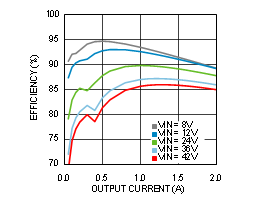Figure 1. Efficiency VOUT = 5 V TA = 25°C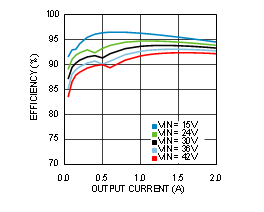Figure 3. Efficiency VOUT = 12 V TA = 25°C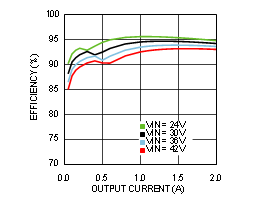Figure 5. Efficiency VOUT = 15 V TA = 25°C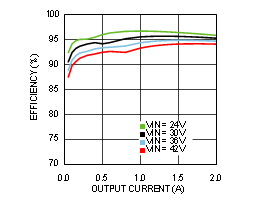Figure 7. Efficiency VOUT = 18 V TA = 25°C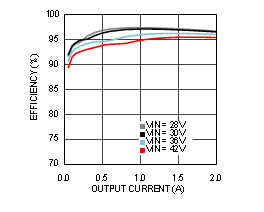Figure 9. Efficiency VOUT = 24 V TA = 25°C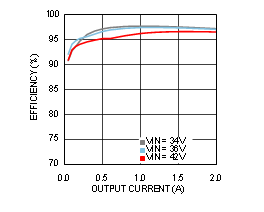Figure 11. Efficiency VOUT = 30 V TA = 25°C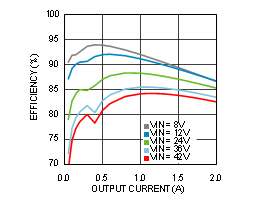Figure 13. Efficiency VOUT = 5 V TA = 85°CFigure 15. Efficiency VOUT = 12 V TA = 85°C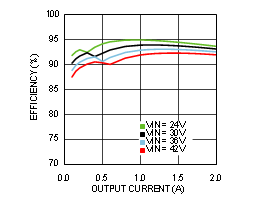Figure 17. Efficiency VOUT = 15 V TA = 85°C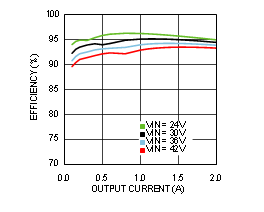Figure 19. Efficiency VOUT = 18 V TA = 85°C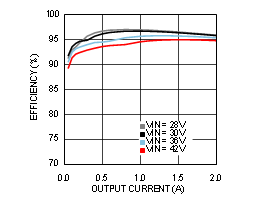Figure 21. Efficiency VOUT = 24 V TA = 85°C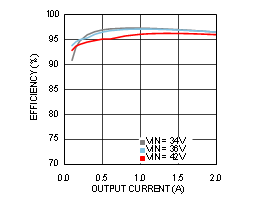Figure 23. Efficiency VOUT = 30 V TA = 85°C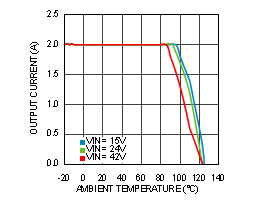Figure 25. Thermal Derating VOUT = 12 V, RθJA = 16°C/W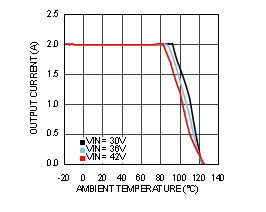Figure 27. Thermal Derating VOUT = 24 V, RθJA = 16°C/W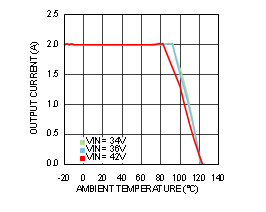Figure 29. Thermal Derating VOUT = 30 V, RθJA = 16°C/W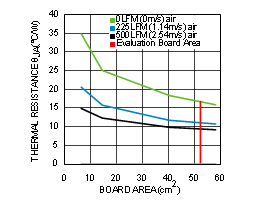Figure 31. Package Thermal Resistance RθJA
4-Layer Printed-Circuit-Board With 1-oz Copper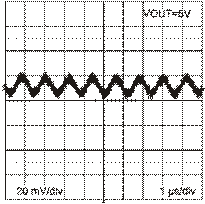Figure 33. Output Ripple
VIN = 12 V, IOUT = 2 A, Ceramic COUT, BW = 200 MHzFigure 35. Load Transient Response VIN = 24 V, VOUT = 12 V
Load Step from 10% to 100%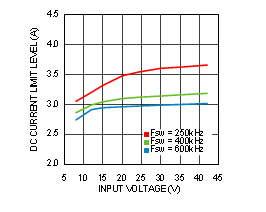Figure 37. Current Limit vs. Input Voltage
VOUT = 5 V TA = 25°C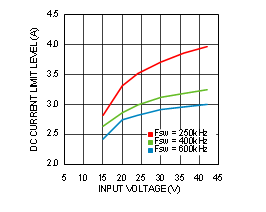Figure 39. Current Limit vs. Input Voltage
VOUT = 12 V TA = 25°C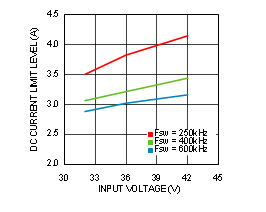Figure 41. Current Limit vs. Input Voltage
VOUT = 24 V TA = 25°C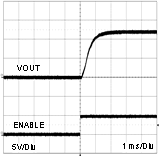Figure 43. Start-Up
VIN = 24 V IOUT = 2 A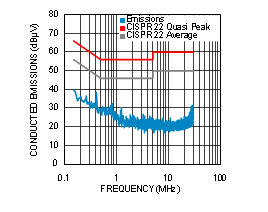Figure 45. Conducted EMI, VOUT = 12 V
Evaluation Board BOM and 3.3-µH 2×10-µF LC line filter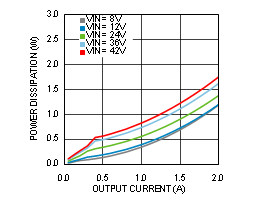Figure 2. Power Dissipation VOUT = 5 V TA = 25°C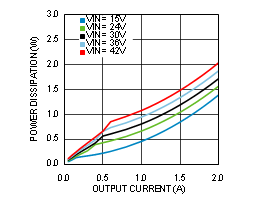Figure 4. Power Dissipation VOUT = 12 V TA = 25°C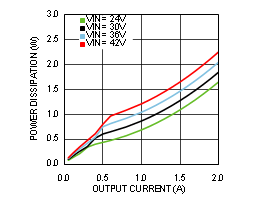Figure 6. Power Dissipation VOUT = 15 V TA = 25°C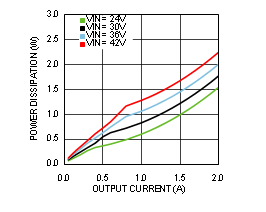Figure 8. Power Dissipation VOUT = 18 V TA = 25°C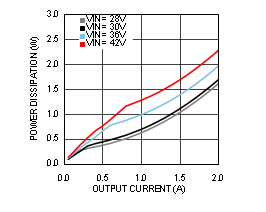Figure 10. Power Dissipation VOUT = 24 V TA = 25°C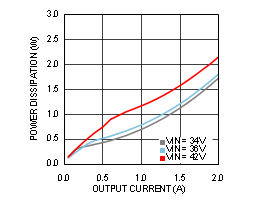Figure 12. Power Dissipation VOUT = 30 V TA = 25°C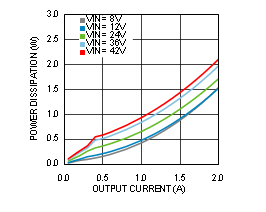Figure 14. Power Dissipation VOUT = 5 V TA = 85°C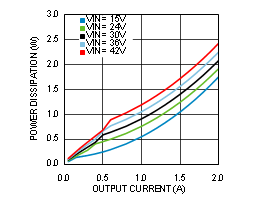Figure 16. Power Dissipation VOUT = 12 V T = 85°C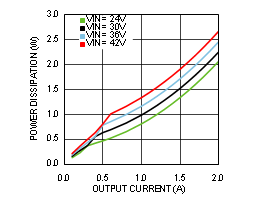Figure 18. Power Dissipation VOUT = 15 V TA = 85°CFigure 20. Power Dissipation VOUT = 18 V TA = 85°C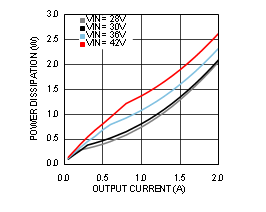Figure 22. Power Dissipation VOUT = 24 V TA = 85°C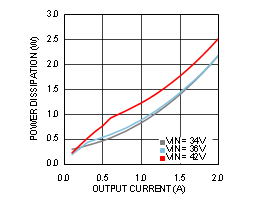Figure 24. Power Dissipation VOUT = 30 V TA = 85°C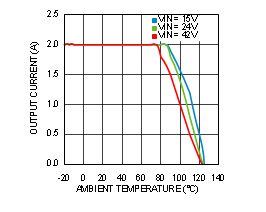Figure 26. Thermal Derating VOUT = 12 V, RθJA = 20°C/W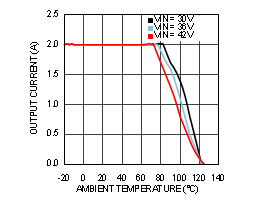Figure 28. Thermal Derating VOUT = 24 V, RθJA = 20°C/WFigure 30. Thermal Derating VOUT = 30 V, RθJA = 20°C/W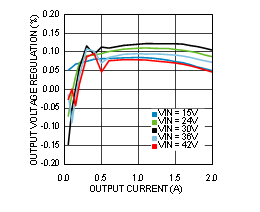Figure 32. Line and Load Regulation TA = 25°C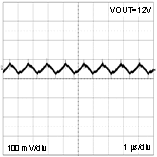Figure 34. Output Ripple
VIN = 24 V, IOUT = 2 A, Polymer Electrolytic COUT, BW = 200 MHz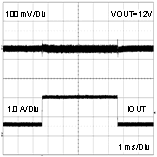Figure 36. Load Transient Response VIN = 24 V, VOUT = 12 V
Load Step from 30% to 100%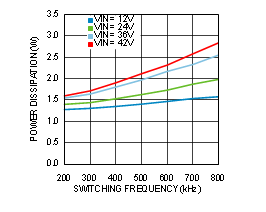Figure 38. Switching Frequency vs. Power Dissipation
VOUT = 5 V TA = 25°C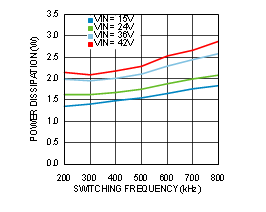Figure 40. Switching Frequency vs. Power Dissipation
VOUT = 12 V TA = 25°C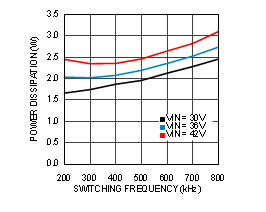Figure 42. Switching Frequency vs. Power Dissipation
VOUT = 24 V TA = 25°C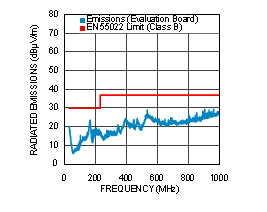Figure 44. Radiated EMI of Evaluation Board, VOUT = 12 V